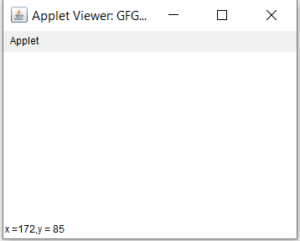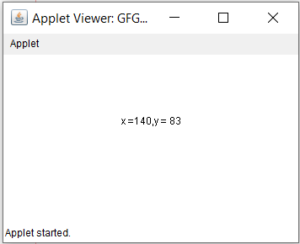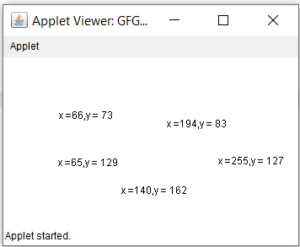# MouseListener and MouseMotionListener in Java

MouseListener and MouseMotionListener is an interface in java.awt.event package . Mouse events
are of two types. MouseListener handles the events when the mouse is not in motion. While MouseMotionListener
handles the events when mouse is in motion.

There are five types of events that MouseListener can generate. There are five abstract functions that represent these five events. The abstract functions are :

1. void mouseReleased(MouseEvent e) : Mouse key is released
2. void mouseClicked(MouseEvent e) : Mouse key is pressed/released
3. void mouseExited(MouseEvent e) : Mouse exited the component
4. void mouseEntered(MouseEvent e) : Mouse entered the component
5. void mousepressed(MouseEvent e) : Mouse key is pressed

There are two types of events that MouseMotionListener can generate. There are two abstract functions that represent these five events. The abstract functions are :

1. void mouseDragged(MouseEvent e) : Invoked when a mouse button is pressed in the component and dragged. Events are passed until the user releases the mouse button.
2. void mouseMoved(MouseEvent e) : invoked when the mouse cursor is moved from one point to another within the component, without pressing any mouse buttons.

The following programs are a illustration of MouseListener and MouseMotionListener.

1. Program to handle MouseListener events

 `// Java program to handle MouseListener events ` `import` `java.awt.*; ` `import` `java.awt.event.*; ` `import` `javax.swing.*; ` `class` `Mouse ``extends` `Frame ``implements` `MouseListener { ` ` `  `    ``// Jlabels to display the actions of events of mouseListener ` `    ``// static JLabel label1, label2, label3; ` ` `  `    ``// default constructor ` `    ``Mouse() ` `    ``{ ` `    ``} ` ` `  `    ``// main class ` `    ``public` `static` `void` `main(String[] args) ` `    ``{ ` `        ``// create a frame ` `        ``JFrame f = ``new` `JFrame(``"MouseListener"``); ` ` `  `        ``// set the size of the frame ` `        ``f.setSize(``600``, ``100``); ` ` `  `        ``// close the frame when close button is pressed ` `        ``f.setDefaultCloseOperation(JFrame.EXIT_ON_CLOSE); ` ` `  `        ``// create anew panel ` `        ``JPanel p = ``new` `JPanel(); ` ` `  `        ``// set the layout of the panel ` `        ``p.setLayout(``new` `FlowLayout()); ` ` `  `        ``// initialize the labels ` `        ``label1 = ``new` `JLabel(``"no event  "``); ` ` `  `        ``label2 = ``new` `JLabel(``"no event  "``); ` ` `  `        ``label3 = ``new` `JLabel(``"no event  "``); ` ` `  `        ``// create an object of mouse class ` `        ``Mouse m = ``new` `Mouse(); ` ` `  `        ``// add mouseListener to the frame ` `        ``f.addMouseListener(m); ` ` `  `        ``// add labels to the panel ` `        ``p.add(label1); ` `        ``p.add(label2); ` `        ``p.add(label3); ` ` `  `        ``// add panel to the frame ` `        ``f.add(p); ` ` `  `        ``f.show(); ` `    ``} ` ` `  `    ``// getX() and getY() functions return the ` `    ``// x and y coordinates of the current ` `    ``// mouse position ` `    ``// getClickCount() returns the number of ` `    ``// quick consecutive clicks made by the user ` ` `  `    ``// this function is invoked when the mouse is pressed ` `    ``public` `void` `mousePressed(MouseEvent e) ` `    ``{ ` ` `  `        ``// show the point where the user pressed the mouse ` `        ``label1.setText(``"mouse pressed at point:"` `                       ``+ e.getX() + ``" "` `+ e.getY()); ` `    ``} ` ` `  `    ``// this function is invoked when the mouse is released ` `    ``public` `void` `mouseReleased(MouseEvent e) ` `    ``{ ` ` `  `        ``// show the point where the user released the mouse click ` `        ``label1.setText(``"mouse released at point:"` `                       ``+ e.getX() + ``" "` `+ e.getY()); ` `    ``} ` ` `  `    ``// this function is invoked when the mouse exits the component ` `    ``public` `void` `mouseExited(MouseEvent e) ` `    ``{ ` ` `  `        ``// show the point through which the mouse exited the frame ` `        ``label2.setText(``"mouse exited through point:"` `                       ``+ e.getX() + ``" "` `+ e.getY()); ` `    ``} ` ` `  `    ``// this function is invoked when the mouse enters the component ` `    ``public` `void` `mouseEntered(MouseEvent e) ` `    ``{ ` ` `  `        ``// show the point through which the mouse entered the frame ` `        ``label2.setText(``"mouse entered at point:"` `                       ``+ e.getX() + ``" "` `+ e.getY()); ` `    ``} ` ` `  `    ``// this function is invoked when the mouse is pressed or released ` `    ``public` `void` `mouseClicked(MouseEvent e) ` `    ``{ ` ` `  `        ``// getClickCount gives the number of quick, ` `        ``// consecutive clicks made by the user ` `        ``// show the point where the mouse is i.e ` `        ``// the x and y coordinates ` `        ``label3.setText(``"mouse clicked at point:"` `                       ``+ e.getX() + ``" "` `                       ``+ e.getY() + ``"mouse clicked :"` `+ e.getClickCount()); ` `    ``} ` `} `

Output :Note : The following program might not run in an online compiler please use an offline IDE

Let’s take another example on MouseListener,the question is:
Q. Write an applet which displays x and y co-ordinate in it’s status bar whenever the user click anywhere in the Applet window.
Ans.
Note: This code is with respect to Netbeans IDE.

 `//Program of an applet which ` `//displays x and y co-ordinate ` `//in it's status bar,whenever  ` `//the user click anywhere in ` `//the applet window. ` ` `  ` `  `import` `java.awt.*; ` `import` `java.awt.event.*; ` `import` `java.applet.*; ` `public` `class` `GFG ``extends` `Applet ``implements` `MouseListener ` `{ ` `public` `void` `init() ` `{ ` `this``.addMouseListener (``this``); ` `//first "this" represent source ` `//(in this case it is applet which ` `//is current calling object) and ` `//second "this" represent  ` `//listener(in this case it is GFG)   ` `} ` `public` `void` `mouseClicked(MouseEvent m) ` `{ ` ` ``int` `x = m.getX(); ` ` ``int` `y = m.getY(); ` ` ``String str = ``"x ="` `+x+``",y = "``+y; ` ` ``showStatus(str); ` `} ` ` `  `    ``@Override` `    ``public` `void` `mousePressed(MouseEvent e) { ` `        `  `} ` ` `  `    ``@Override` `    ``public` `void` `mouseReleased(MouseEvent e) { ` `        `  `    ``} ` ` `  `    ``@Override` `    ``public` `void` `mouseEntered(MouseEvent e) { ` `         `  `    ``} ` ` `  `    ``@Override` `    ``public` `void` `mouseExited(MouseEvent e) { ` `        `  `    ``} ` `} `

Output:Output showing (x,y) in status bar

Modification: Now our aim is to improve above program so that co-ordinates should display at that point only where click has been made

Note: This code is with respect to Netbeans IDE.

 `//Co-ordinates should display ` `//at that point only wherever ` `//their is click on canvas ` ` `  `import` `java.awt.*; ` `import` `java.awt.event.*; ` `import` `java.applet.*; ` `public` `class` `GFG ``extends` `Applet ``implements` `MouseListener ` `{ ` `private` `int` `x,y; ` `private` `String str = ``" "``; ` `public` `void` `init() ` `{ ` `this``.addMouseListener (``this``); ` `//first "this" represent source ` `//(in this case it is applet which ` `// is current calling object) and ` `// second "this" represent listener ` `//(in this case it is GFG)   ` `} ` `public` `void` `paint(Graphics g) ` `{ ` `g.drawString(str,x,y); ` `} ` `public` `void` `mouseClicked(MouseEvent m) ` `{ ` `  ``x = m.getX(); ` `  ``y = m.getY(); ` `  ``str = ``"x ="` `+x+``",y = "``+y; ` `repaint(); ``// we have made this  ` `//call because repaint() will ` `//call paint() method for us. ` `//If we comment out this line, ` `//then we will see output  ` `//only when focus is on the applet ` `//i.e on maximising the applet window ` `//because paint() method is called ` `//when applet screen gets the focus. ` `//repaint() is a method of Component ` `//class and prototype for this method is: ` `//public void repaint() ` ` `  `} ` ` `  ` ``public` `void` `mouseEntered(MouseEvent m) ` `//over-riding all the methods given by ` `// MouseListener ` `{ ` `} ` ` ``public` `void` `mouseExited(MouseEvent m) ` `{ ` `} ` ` ``public` `void` `mousePressed(MouseEvent m) ` `{ ` `} ` ` ``public` `void` `mouseReleased(MouseEvent m) ` `{ ` `} ` `}  `

OutputOutput showing (x,y) in canvas

Now one more unusual thing will come in output which is that, we will not able to see previous co-ordinates.But why?
In Java, before calling paint() method, it calls one more method which is update() and it do the following things:

1. it repaints the applet background with current color.
2. it then calls paint().

Now to see previous co-ordinates as well:
we have to over-ride update() method also and it’s prototype is similar to paint().

Further Modification To see previous co-ordinates as well:

Note: This code is with respect to Netbeans IDE.

 `//Co-ordinates should display ` `//at that point only wherever ` `//their is click on canvas and also  ` `//able to see the previous coordinates  ` ` `  `import` `java.awt.*; ` `import` `java.awt.event.*; ` `import` `java.applet.*; ` `public` `class` `GFG ``extends` `Applet ``implements` `MouseListener ` `{ ` `private` `int` `x,y; ` `private` `String str = ``" "``; ` `public` `void` `init() ` `{ ` `this``.addMouseListener (``this``);  ` `} ` `public` `void` `paint(Graphics g) ` `{ ` `g.drawString(str,x,y); ` `} ` `public` `void` `update(Graphics g) ` `{ ` `paint(g); ` `} ` `public` `void` `mouseClicked(MouseEvent m) ` `{ ` `  ``x = m.getX(); ` `  ``y = m.getY(); ` `  ``str = ``"x ="` `+x+``",y = "``+y; ` `repaint(); ` `} ` ` ``public` `void` `mouseEntered(MouseEvent m) ` `{ ` `} ` ` ``public` `void` `mouseExited(MouseEvent m) ` `{ ` `} ` ` ``public` `void` `mousePressed(MouseEvent m) ` `{ ` `} ` ` ``public` `void` `mouseReleased(MouseEvent m) ` ` ``{      ` ` ``} ` `} `

OutputOutput showing previous co-ordinate as well

2. Program to handle MouseMotionListener events

 `// Java Program to illustrate MouseMotionListener events ` `import` `java.awt.*; ` `import` `java.awt.event.*; ` `import` `javax.swing.*; ` `class` `Mouse ``extends` `Frame ``implements` `MouseMotionListener { ` ` `  `    ``// Jlabels to display the actions of events of MouseMotionListener ` `    ``static` `JLabel label1, label2; ` ` `  `    ``// default constructor ` `    ``Mouse() ` `    ``{ ` `    ``} ` ` `  `    ``// main class ` `    ``public` `static` `void` `main(String[] args) ` `    ``{ ` `        ``// create a frame ` `        ``JFrame f = ``new` `JFrame(``"MouseMotionListener"``); ` ` `  `        ``// set the size of the frame ` `        ``f.setSize(``600``, ``400``); ` ` `  `        ``// close the frame when close button is pressed ` `        ``f.setDefaultCloseOperation(JFrame.EXIT_ON_CLOSE); ` ` `  `        ``// create anew panel ` `        ``JPanel p = ``new` `JPanel(); ` ` `  `        ``// set the layout of the panel ` `        ``p.setLayout(``new` `FlowLayout()); ` ` `  `        ``// initialize the labels ` `        ``label1 = ``new` `JLabel(``"no event  "``); ` ` `  `        ``label2 = ``new` `JLabel(``"no event  "``); ` ` `  `        ``// create an object of mouse class ` `        ``Mouse m = ``new` `Mouse(); ` ` `  `        ``// add mouseListener to the frame ` `        ``f.addMouseMotionListener(m); ` ` `  `        ``// add labels to the panel ` `        ``p.add(label1); ` `        ``p.add(label2); ` ` `  `        ``// add panel to the frame ` `        ``f.add(p); ` ` `  `        ``f.show(); ` `    ``} ` ` `  `    ``// getX() and getY() functions return the ` `    ``// x and y coordinates of the current ` `    ``// mouse position ` ` `  `    ``// invoked when mouse button is pressed ` `    ``// and dragged from one point to another ` `    ``// in a  component ` `    ``public` `void` `mouseDragged(MouseEvent e) ` `    ``{ ` `        ``// update the label to show the point ` `        ``// through which point mouse is dragged ` `        ``label1.setText(``"mouse is dragged through point "` `                       ``+ e.getX() + ``" "` `+ e.getY()); ` `    ``} ` ` `  `    ``// invoked when the cursor is moved from ` `    ``// one point to another within the component ` `    ``public` `void` `mouseMoved(MouseEvent e) ` `    ``{ ` `        ``// update the label to show the point to which the cursor moved ` `        ``label2.setText(``"mouse is moved to point "` `                       ``+ e.getX() + ``" "` `+ e.getY()); ` `    ``} ` `} `

Output :3. Java program to illustrate MouseListener and MouseMotionListener events
simultanously

 `// Java program to illustrate MouseListener  ` `// and MouseMotionListener events ` `// simultaneously ` ` `  `import` `java.awt.*; ` `import` `java.awt.event.*; ` `import` `javax.swing.*; ` `class` `Mouse ``extends` `Frame ``implements` `MouseMotionListener, MouseListener { ` ` `  `    ``// Jlabels to display the actions of events of MouseMotionListener and MouseListener ` `    ``static` `JLabel label1, label2, label3, label4, label5; ` ` `  `    ``// default constructor ` `    ``Mouse() ` `    ``{ ` `    ``} ` ` `  `    ``// main class ` `    ``public` `static` `void` `main(String[] args) ` `    ``{ ` `        ``// create a frame ` `        ``JFrame f = ``new` `JFrame(``"MouseListener and MouseMotionListener"``); ` ` `  `        ``// set the size of the frame ` `        ``f.setSize(``900``, ``300``); ` ` `  `        ``// close the frame when close button is pressed ` `        ``f.setDefaultCloseOperation(JFrame.EXIT_ON_CLOSE); ` ` `  `        ``// create anew panel ` `        ``JPanel p = ``new` `JPanel(); ` `        ``JPanel p1 = ``new` `JPanel(); ` ` `  `        ``// set the layout of the frame ` `        ``f.setLayout(``new` `FlowLayout()); ` ` `  `        ``JLabel l1, l2; ` ` `  `        ``l1 = ``new` `JLabel(``"MouseMotionListener events  :"``); ` ` `  `        ``l2 = ``new` `JLabel(``"MouseLIstener events  :"``); ` ` `  `        ``// initialize the labels ` `        ``label1 = ``new` `JLabel(``"no event  "``); ` ` `  `        ``label2 = ``new` `JLabel(``"no event  "``); ` ` `  `        ``label3 = ``new` `JLabel(``"no event  "``); ` ` `  `        ``label4 = ``new` `JLabel(``"no event  "``); ` ` `  `        ``label5 = ``new` `JLabel(``"no event  "``); ` ` `  `        ``// create an object of mouse class ` `        ``Mouse m = ``new` `Mouse(); ` ` `  `        ``// add mouseListener and MouseMotionListenerto the frame ` `        ``f.addMouseMotionListener(m); ` `        ``f.addMouseListener(m); ` ` `  `        ``// add labels to the panel ` `        ``p.add(l1); ` `        ``p.add(label1); ` `        ``p.add(label2); ` `        ``p1.add(l2); ` `        ``p1.add(label3); ` `        ``p1.add(label4); ` `        ``p1.add(label5); ` ` `  `        ``// add panel to the frame ` `        ``f.add(p); ` `        ``f.add(p1); ` ` `  `        ``f.show(); ` `    ``} ` ` `  `    ``// getX() and getY() functions return the ` `    ``// x and y coordinates of the current ` `    ``// mouse position ` `    ``// getClickCount() returns the number of ` `    ``// quick consecutive clicks made by the user ` ` `  `    ``// MouseMotionListener events ` ` `  `    ``// invoked when mouse button is pressed ` `    ``// and dragged from one point to another ` `    ``// in a  component ` `    ``public` `void` `mouseDragged(MouseEvent e) ` `    ``{ ` `        ``// update the label to show the point ` `        ``// through which point mouse is dragged ` `        ``label1.setText(``"mouse is dragged through point "` `                       ``+ e.getX() + ``" "` `+ e.getY()); ` `    ``} ` ` `  `    ``// invoked when the cursor is moved from ` `    ``// one point to another within the component ` `    ``public` `void` `mouseMoved(MouseEvent e) ` `    ``{ ` `        ``// update the label to show the point to which the cursor moved ` `        ``label2.setText(``"mouse is moved to point "` `                       ``+ e.getX() + ``" "` `+ e.getY()); ` `    ``} ` ` `  `    ``// MouseListener events ` ` `  `    ``// this function is invoked when the mouse is pressed ` `    ``public` `void` `mousePressed(MouseEvent e) ` `    ``{ ` ` `  `        ``// show the point where the user pressed the mouse ` `        ``label3.setText(``"mouse pressed at point:"` `                       ``+ e.getX() + ``" "` `+ e.getY()); ` `    ``} ` ` `  `    ``// this function is invoked when the mouse is released ` `    ``public` `void` `mouseReleased(MouseEvent e) ` `    ``{ ` ` `  `        ``// show the point where the user released the mouse click ` `        ``label3.setText(``"mouse released at point:"` `                       ``+ e.getX() + ``" "` `+ e.getY()); ` `    ``} ` ` `  `    ``// this function is invoked when the mouse exits the component ` `    ``public` `void` `mouseExited(MouseEvent e) ` `    ``{ ` ` `  `        ``// show the point through which the mouse exited the frame ` `        ``label4.setText(``"mouse exited through point:"` `                       ``+ e.getX() + ``" "` `+ e.getY()); ` `    ``} ` ` `  `    ``// this function is invoked when the mouse enters the component ` `    ``public` `void` `mouseEntered(MouseEvent e) ` `    ``{ ` ` `  `        ``// show the point through which the mouse entered the frame ` `        ``label4.setText(``"mouse entered at point:"` `                       ``+ e.getX() + ``" "` `+ e.getY()); ` `    ``} ` ` `  `    ``// this function is invoked when the mouse is pressed or released ` `    ``public` `void` `mouseClicked(MouseEvent e) ` `    ``{ ` ` `  `        ``// getClickCount gives the number of quick, ` `        ``// consecutive clicks made by the user ` `        ``// show the point where the mouse is i.e ` `        ``// the x and y coordinates ` `        ``label5.setText(``"mouse clicked at point:"` `                       ``+ e.getX() + ``" "` `                       ``+ e.getY() + ``"mouse clicked :"` `+ e.getClickCount()); ` `    ``} ` `} `

output :MouseListener vs MouseMotionListener

• MouseListener: MouseListener events are invoked when the mouse is not in motion and is stable . It generates events such as mousePressed, mouseReleased, mouseClicked, mouseExited and mouseEntered (i.e when the mouse buttons are pressed or the mouse enters or exits the component). The object of this class must be registered with the component and they are registered using addMouseListener() method.
• MouseMotionListener: MouseMotionListener events are invoked when the mouse is in motion . It generates events such as mouseMoved and mouseDragged (i.e when the mouse is moved from one point to another within the component or the mouse button is pressed and dragged from one point to another ). The object of this class must be registered with the component and they are registered using addMouseMotionListener() method.

My Personal Notes arrow_drop_upCheck out this Author's contributed articles.

If you like GeeksforGeeks and would like to contribute, you can also write an article using contribute.geeksforgeeks.org or mail your article to contribute@geeksforgeeks.org. See your article appearing on the GeeksforGeeks main page and help other Geeks.

Please Improve this article if you find anything incorrect by clicking on the "Improve Article" button below.

Improved By : AnshulVaidya, Akanksha_Rai

Article Tags :
Practice Tags :

2

Please write to us at contribute@geeksforgeeks.org to report any issue with the above content.